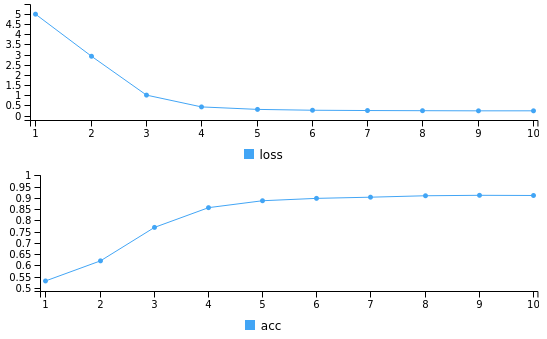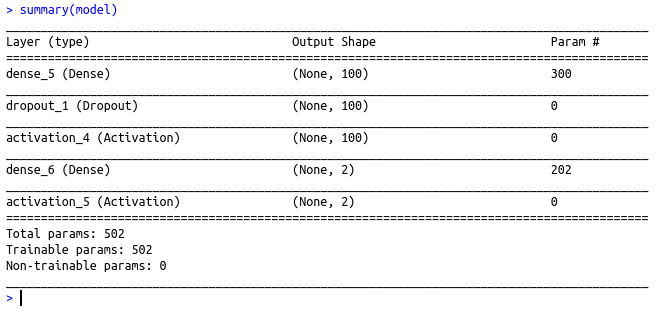• #5, First Floor, 4th Street Dr. Subbarayan Nagar Kodambakkam, Chennai-600 024 Landmark : Samiyar Madam
• pro@slogix.in
• +91- 81240 01111

### How to perform Logistic regression in R using Deep Neural network?

###### Description

To perform logistic regression in R using deep neural network

h4>Functions Used

model layer_dense() – Add a input,output or hidden layer

layer_activation() – Activation function for the layer

compile() – To compile the model

fit() – To fit the model using the train set

predict() – To predict using the test set

#### Libraries Required :

library(keras)

###### Process

Convert the categorical variables to equivalent numeric classes

Split the data set as train set and test set

Initialize the keras sequential model

Build the model with input layers,hidden layers and output layer as per the data size along with the activation function(here 2 I/P layer with relu activation and 2 O/P layer with sigmoid activation)

Compile the model with required loss,metrics and optimizer(here loss=binary_crossentropy,optimizer=adam,metrics=accuracy)

Fit the model using the train set

Predict using the test set

Evaluate the metrics

###### Sapmle Code

library(caret)
library(keras)
#Setting seed to reproduce results of random sampling data
set.seed(100)
#Train set
train_ind<-createDataPartition(data\$Gender,p=0.8,list=FALSE)
train_data<-data[train_ind,]
xtrain=train_data[,2:3]
ytrain=train_data\$Gender
ytrain=factor(ytrain,labels=c(0,1))
#Test set
test=data[-train_ind,]
xtest=test[,2:3]
ytest=factor(test\$Gender,labels=c(0,1))

#converting the target variable to once hot encoded vectors using keras inbuilt function
train_y<-to_categorical(ytrain,2)
#defining a keras sequential model
model %
layer_dense(units = 100, input_shape = 2) %>%
layer_dropout(rate=0.4)%>%
layer_activation(activation = ‘relu’) %>%
layer_dense(units = 2)%>%
layer_activation(activation= “sigmoid”)
#compiling the defined model with metric = accuracy and optimiser as adam.
model %>% compile(
loss = ‘binary_crossentropy’,
metrics = ‘accuracy’
)
#Summary of the model
summary(model)
class(ytrain)
#fitting the model on the training dataset
model %>% fit(as.matrix(xtrain), train_y, epochs = 10, batch_size = 128)

#Predict using the Test data
yt=predict_classes(model,as.matrix(xtest))
cat(“The confusion matrix is \n”)
print(confusionMatrix(as.factor(yt),as.factor(ytest)))

###### Screenshots